# High School Chemistry : Compounds and Bonding

## Example Questions

← Previous 1 3 4 5 6 7

### Example Question #1 : Compounds And Bonding

Which answer choice shows the correct chemical formula for barium sulfate?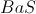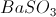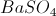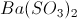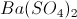Explanation:

We should first remember the difference between sulfate, sulfite, and sulfide. Sulfate is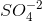, sulfite is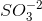` and sulfide is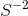.

The only answer choices that could be right must havein them. We then need to see that barium usually has a charge of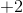, as the periodic table shows us, and so we need a charge ofto cancel that out. The answer is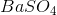.

### Example Question #2 : Naming Compounds

Which of the following chemical compounds is written incorrectly?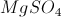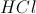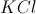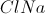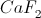Explanation:

Ionic chemical compounds are always named with the metal before the non-metal. The correct way to name sodium chloride would be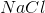, and not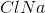, because sodium is the metal and chloride is the non-metal.

All the other examples have the metal before the non-metal. The other answer choices correspond to potassium chloride, magnesium sulfate, hydrogen chloride (hydrochloric acid), and calcium fluoride.

### Example Question #1 : Compounds And Bonding

Name the following compound: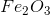Iron trioxide

Iron(II) oxide

Iron oxide

Iron(III) oxide

Iron(III) oxide

Explanation:

Because iron has multiple oxidation states, we need to follow the cation with the roman numeral that signifies how many electrons each iron ion has donated to oxygen. Since oxygen gains two electrons in order to satisfy its octet, the three oxygens will accept a total of six electrons from two iron atoms. This means that each iron must donate three electrons, so that each atom donates electrons equally. As a result, the name of the ionic compound is iron(III) oxide.

Another acceptable name for the compound would be ferric oxide. The term "ferric" refers to iron(III) and the term "ferrous" refers to iron (II).

### Example Question #3 : Naming Compounds

Name the following compound: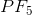Monophosphorus pentafluoride

Phosphorus pentafluoride

Phosphorus fluoride

Phosphorus tetrafluoride

Phosphorus pentafluoride

Explanation:

When naming covalent compounds, it is important to use a prefix before each element in order to designate how many atoms are in the compound. One key exception is when you only have one atom at the beginning of the compound (such as in this question). You will never start a covalent compound with "mono-". The prefix for five is "penta-", so the name of this covalent compound is phosphorus pentafluoride. The name indicates that there is one phosphorus atom bound to five fluorine atoms.

### Example Question #51 : Elements And Compounds

Which of the following molecular formulas is written incorrectly?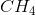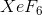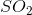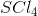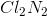Explanation:

When naming molecular compounds, the rule is to put the element farthest to the left of the period table and closest to the bottom of the periodic table first.

For example, in sulfur dioxide, since sulfur and oxygen are both in group 6A, we put sulfur first since it is below oxygen in the group:. Similarly, in carbon dioxide we put carbon first because it is farther to the left in the period: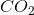.is written incorrectly because nitrogen is farther left on the periodic table than chlorine. Written correctly, this compound would be dinitrogen dichloride: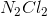.

### Example Question #1 : Compounds And Bonding

Choose the correct name for the following compound.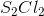Sulfur dichloride

Diasulfur diachloride

Sulfur chlorate

Disulfur dichloride

Sulfur chloride

Disulfur dichloride

Explanation:

The moleculeis a molecular compound because it contains two non-metals; therefore, it contains no ionic bonds. We use prefixes to describe the subscript for each element in molecular compounds. The molecule in this question has a subscript of two for each of the elements, so the prefix di- should be added to both sulfur and chlorine.

For molecular compounds, we change the ending of the final element to -ide, giving us the name "disulfur dichloride."

It is important to note that "chlorate" has a separate meaning, and refers to the complex ion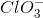.

### Example Question #1 : Compounds And Bonding

Choose the formula that corresponds to sodium oxide.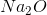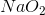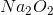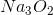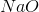Explanation:

We know that sodium oxide is a binary ionic compound because it contains one metal cation and one non-metal anion. To write the ionic formula we must write the cation first, followed by the anion, and make sure the net charge of the molecule is zero.

From the periodic table, we know that sodium (Na) has a charge of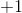and oxygen (O) has a charge of. We know that the final charge on the molecule must be zero. To balance the charge, there must be two sodium atoms for every oxygen atom.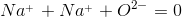The other answer choices do not have a net charge of zero.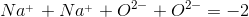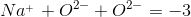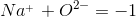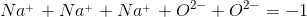### Example Question #8 : Naming Compounds

Which of the following ionic compounds is written incorrectly?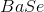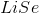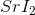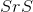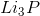Explanation:

Ionic compounds are written with the cation (metal) first, followed by the anion (non-metal). All of the answer choices satisfy this requirement.

Next, we must look at the net charge on each molecule. All of the ionic compounds have a net charge of zero except for, which has a charge ofas written.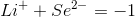The correct ionic formula for a compound containing these two elements is: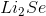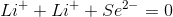### Example Question #1 : Compounds And Bonding

Name the compound  and determine the type of molecule.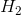Hydrogen; binary molecular compound

Hydrogen; diatomic molecular compound

Hydrogen monohydride; diatomic molecular compound

This molecule cannot exist

Hydrogen monohydride; binary ionic compound

Hydrogen; diatomic molecular compound

Explanation:

The given compound is the formula for gaseous hydrogen. Hydrogen atoms have only one valence electron and will form covalent bonds with other hydrogen atoms to fill the s orbital, generating a covalent bond. This tells us that it is a molecular bond, since no ionic bonds are present.

Binary compounds always contain two elements. This compound contains only one element, and cannot be considered binary. Instead, it is referred to as "diatomic," meaning that the molecule is composed of two atoms of the same element. Most diatomic molecules are gaseous, and include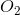,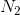, and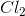(among others). The noble gases will not form diatomic particles.

Naming diatomic molecules is simple, since there is only one element involved. Most commonly,is simply named hydrogen or gaseous hydrogen. Keep this in mind when working with reactions. If a reaction occurs between nitrogen and oxygen, it is occurring between their diatomic molecules, not the individual atoms themselves.

### Example Question #1 : Compounds And Bonding

Which of the following statements is false regarding the nomenclature and formulas of binary molecular compounds?

The prefix mono- should only be used on the second element

The ending of the second element is replaced with the suffix -ide

Molecular compounds can contain only non-metals

The prefix hepta- means seven

The element farthest to the left in the periodic table and closer to the top of a group appears first in the name and formula

The element farthest to the left in the periodic table and closer to the top of a group appears first in the name and formula

Explanation:

Binary molecular compounds contain two non-metal elements joined by covalent bonds. Conventions for naming these compounds require that prefixes be used to denote the subscript of each element in the compound, telling the number of atoms in the name. The prefix for one, mono-, is only applied to the second element in the name, assuming that it has no subscript (one atom per molecule). The suffix -ide is attached to the second element in the compound to complete the name.

When determining which element should be first, you must reference the periodic table. Elements located more to the left and toward the bottom of the non-metals will appear first in the compound name. Carbon monoxide is a simple example of the leftmost element coming first, while bromine monochloride is an example of the bottommost element coming first.

The following is a list of prefixes used in naming molecular compounds:

One: mono-

Two: di-

Three: tri-

Four: tetra-

Five: penta-

Six: hexa-

Seven: hepta-

Eight: octa-

Nine: nona-

Ten: deca-

← Previous 1 3 4 5 6 7

### All High School Chemistry Resources